Related Articles
Python | Pandas TimedeltaIndex.difference
• Last Updated : 29 Dec, 2018

Python is a great language for doing data analysis, primarily because of the fantastic ecosystem of data-centric python packages. Pandas is one of those packages and makes importing and analyzing data much easier.

Pandas` TimedeltaIndex.difference()` function return a new Index with elements from the index that are not in other. This is the set difference of two Index objects. It’s sorted if sorting is possible.

Syntax : TimedeltaIndex.difference(other)

Parameters :
other : Index or array-like

Return : difference : Index

Example #1: Use `TimedeltaIndex.difference()` function to find the set difference of two TimedeltaIndex objects.

 `# importing pandas as pd``import` `pandas as pd`` ` `# Create the first TimedeltaIndex object``tidx1 ``=` `pd.TimedeltaIndex(data ``=``[``'06:05:01.000030'``, ``'+23:59:59.999999'``, ``                                 ``'22 day 2 min 3us 10ns'``, ``'+23:29:59.999999'``, ``                                                         ``'+12:19:59.999999'``])`` ` `# Create the seccond TimedeltaIndex object``tidx2 ``=` `pd.TimedeltaIndex(data ``=``[``'09:11:18.000030'``, ``'+23:59:59.999999'``,``                                 ``'9 day 18 min 3us '``, ``'+23:29:59.999999'``,``                                                     ``'+12:19:59.999999'``])`` ` `# Print the first and second TimedeltaIndex object``print``(tidx1, ``"\n"``, tidx2)`

Output :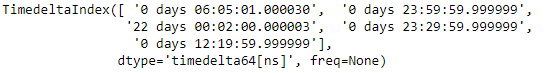Now we will use the `TimedeltaIndex.difference()` function to find the set difference.

 `# find the set difference``tidx1.difference(tidx2)`

Output :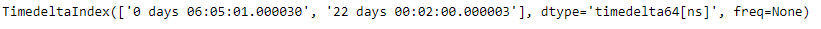As we can see in the output, the `TimedeltaIndex.difference()` function has returned a new object which contains only those elements which are unique to tidx1.

Example #2: Use `TimedeltaIndex.difference()` function to find the set difference of two TimedeltaIndex objects.

 `# importing pandas as pd``import` `pandas as pd`` ` `# Create the first TimedeltaIndex object``tidx1 ``=` `pd.TimedeltaIndex(start ``=``'1 days 02:00:12.001124'``, periods ``=` `5``,``                                              ``freq ``=``'D'``, name ``=``'Koala'``)`` ` `# Create the second TimedeltaIndex object``tidx2 ``=` `pd.TimedeltaIndex(start ``=``'3 days 02:00:12.001124'``, periods ``=` `5``,``                                              ``freq ``=``'D'``, name ``=``'Koala'``)`` ` `# Print the first and second TimedeltaIndex object``print``(tidx1, ``"\n"``, tidx2)`

Output :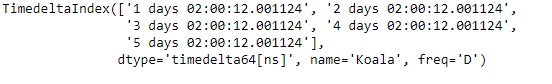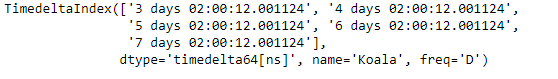Now we will use the `TimedeltaIndex.difference()` function to find the set difference.

 `# find the set difference``tidx1.difference(tidx2)`

Output :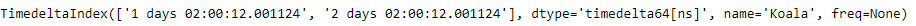As we can see in the output, the `TimedeltaIndex.difference()` function has returned a new object which contains only those elements which are unique to tidx1.

Attention geek! Strengthen your foundations with the Python Programming Foundation Course and learn the basics.

To begin with, your interview preparations Enhance your Data Structures concepts with the Python DS Course.

My Personal Notes arrow_drop_up